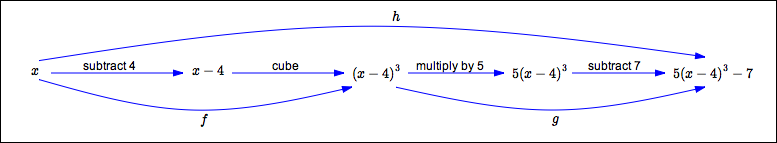﻿ Writing a Function as a Composition

# WRITING A FUNCTION AS A COMPOSITION

• PRACTICE (online exercises and printable worksheets)

When you're given a multi-step task to perform, you may want to break it into pieces,
and assign different pieces to different people.

When you're given a function that does several things,
you may want to break it into ‘smaller’ functions that accomplish the same job!

## EXAMPLE (breaking a composite function into pieces)

Consider the function $\,h(x) = 5(x-4)^3 - 7\,$.
This function $\,h\,$ does the following:

1. subtracts $\,4\,$
2. cubes the result
3. multiplies by $\,5\,$
4. subtracts $\,7\,$
Break these four tasks into two pieces, as shown in the mapping diagram below:• assign the first two tasks (subtract $\,4\,$, then cube) to $\,f\$:   $f(x) = (x-4)^3$
• assign the last two tasks (multiply by $\,5\,$, then subtract $\,7\,$) to $\,g\$:   $g(x) = 5x - 7$
With these assignments, the composite function $\,g\circ f\$ (where $\,f\,$ acts first, followed by $\,g\,$) accomplishes the same thing as $\,h\,$: $$(g\circ f\,)(x) = g(f(x)) = g\bigl((x-4)^3\bigr) = 5(x-4)^3 - 7 = h(x)$$

## You can break a task into pieces in different ways!

Of course, you can delegate the responsibilities in different ways:

• $f\,$ takes the first step (subtract $4$):   $f(x) = x-4$
$g\,$ takes the other three steps (cube, multiply by $5$, subtract $7$):   $g(x) = 5x^3 - 7$
• $f\,$ takes the first three steps (subtract $4$, cube, multiply by $5$):   $f(x) = 5(x-4)^3$
$g\,$ takes the last (subtract $7$):   $g(x) = x - 7$
In both cases, be sure to check that $\,(g\circ f\,)(x) = h(x)\,$.

## You can use more than two helpers!

Or, you can use more ‘helper’ functions.
For example:

• let $\ a\$ subtract $4$:   $a(x) = x-4$
• let $\ b\$ cube:   $b(x) = x^3$
• let $\ c\$ multiply by $5$ and subtract $7$:   $c(x) = 5x - 7$
Then,

\displaystyle \begin{align} (c\circ b\circ a\,)(x) &= c(b(a(x)))\cr &= c(b(x-4))\cr &= c((x-4)^3)\cr &= 5(x-4)^3 - 7\cr &= h(x) \end{align}

The exercises in this lesson give you practice with this process of writing a function as a composition.

Master the ideas from this section